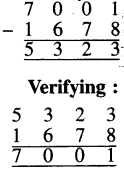# PSEB 4th Class Maths Solutions Chapter 2 Fundamental Operations on Numbers Ex 2.1

Punjab State Board PSEB 4th Class Maths Book Solutions Chapter 2 Fundamental Operations on Numbers Ex 2.1 Textbook Exercise Questions and Answers.

## PSEB Solutions for Class 4 Maths Chapter 2 Fundamental Operations on Numbers Ex 2.1

Question 1.
Add 4 and 2 on a number line.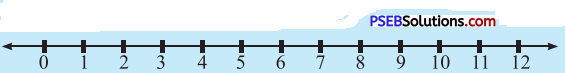Solution: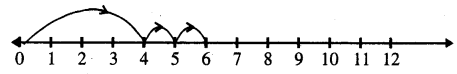1. First of all put a mark on 4 on number line.
2. To add 2, go from left to right one by one two steps.
3. Now we will reach on 6 and this is our answer.
∴ 4 + 2 = 6.Question 2.
Add 6 and 4 on a number line.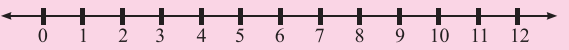Solution: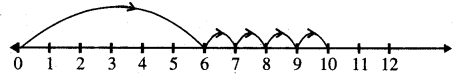1. First of all put a mark on 6 on number line.
2. To add 4, go from left to right one by one four steps.
3. Now, we will reach on 10 and this is our answer.
∴ 6 + 4 = 10.

Question 3.
Subtract 2 from 6 on number line.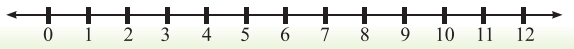Solution: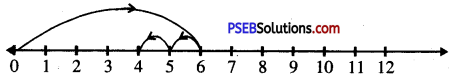1. First of all put a mark on 6 on number line.
2. To subtract 2, go back from right to left one by one two steps.
3. Now, we will reach on 4 and this our answer.
∴ 6 – 2 = 4.Question 4.
Subtract 6 from 11 on a number line.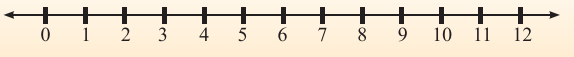Solution: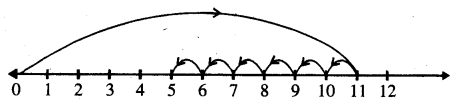1. First of all pur a mark on 11 on the number line.
2. To subtract 6, go back from right to left, one by one, six steps.
3. Now, we will reach on 5 and this is our answer.
∴ 11 – 6 = 5.

5. Solve the following :

Question 1.
374 + 202
Solution: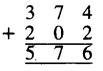Question 2.
356 + 122
Solution: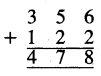Question 3.
4251 + 1244
Solution: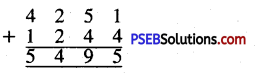Question 4.
7000 + 1789
Solution: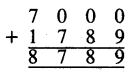Question 5.
999 – 234
Solution: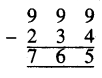Question 6.
798 – 130
Solution: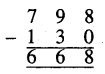Question 7.
9825 – 1214
Solution: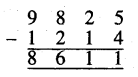Question 8.
7896 – 1234.
Solution: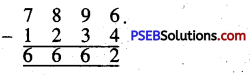6. Solve the following:

Question 1.
769 + 584
Solution: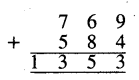Question 2.
649 + 161
Solution: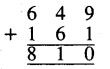Question 3.
3009 + 5691
Solution: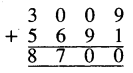Question 4.
2347 + 7437
Solution: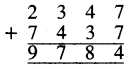Question 5.
769 + 444 + 325
Solution: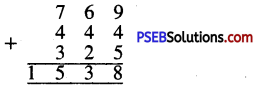Question 6.
688 + 100 + 135
Solution: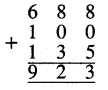Question 7.
2807 + 5938 + 1238
Solution: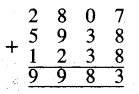Question 8.
7644 + 166 + 1234
Solution: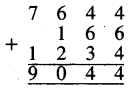Question 9.
768 – 119
Solution: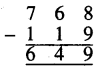Question 10.
6307 – 4156
Solution: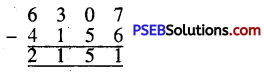Question 11.
7503 – 1219
Solution: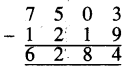Question 12.
7000 – 1234.
Solution: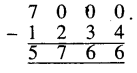Question 1.
7610 – 1733
Solution: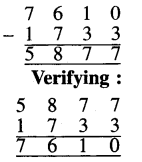Question 2.
6113 – 1167
Solution: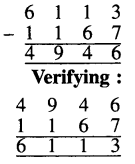Question 3.
6501 – 1212
Solution: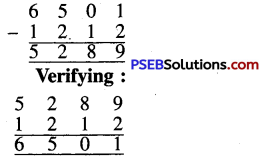Question 4.
4368 – 1239
Solution: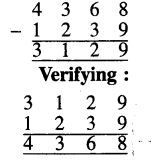Question 5.
7001 – 1678.
Solution: